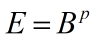Request a Tool

Exponents Calculator

Exponents Calculator is use to calculate exponential value from base and exponent.

Exponential
0

Formula• E = Exponent
• B = Value of base
• p = Value of power

Defination / Uses

The number of times a number is multiplied by itself is referred to as an exponent. For instance, 2 to the third (written as 23) is 2 x 2 x 2 = 8.

This is an exponents calculator that you may use online. Calculate the power of real numbers and huge base integers. Numbers to the power of huge exponents less than 2000, negative exponents, and real numbers or decimals for exponents can all be calculated. Exponentiation is a mathematical process that raises a number called the base to a power called the exponent. If the base is b and the exponent is e, the exponentiation of b by e will be. Our is exactly what this online exponent calculator does for you. The procedure is equal to multiplying the base by itself e times when the exponent is a positive integer.